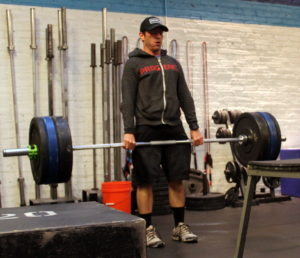## Monday, May 15, 2017

### Monday, May 15, 2017as many rounds as possible in 20 minutes:
5x strict ring dip @ 25/15-lbs.
5x ring dip
25x squat
50x double underas many rounds as possible in 20 minutes:
10x bench dip
25x squat
400m run

Post score to comments.

1.–Scores–
Matt H.: 6 rounds, 135 (sub: 7x weighted strict + 20x sit-up)
Anna G.: 6 rounds, 35 (sub: 7x weighted strict + 20x sit-up)
Neil A.: 4 rounds, 200 (sub: 3x strict + 5x ring dip)
Alex M.: 7 rounds (sub: 3x strict + 5x ring dip)
Kevin K.: 5 rounds (sub: 3x strict + 5x ring dip)
Charles B.: 5 rounds (sub: 3x strict + 5x ring dip, double under practice)
Blaine P.: 6 rounds (sub: 10x squat + 15x lunge)
Teal B.: 3 rounds, 347 (sub: 10x modified ring dip)
Liz L.: 5 rounds, 47 (sub: 10x modified ring dip)
Megan K.: 4 rounds, 218 (sub: 10x modified ring dip)
Mary R.: 5 rounds, 5 (sub: 10x modified ring dip)
Hari P.: 4 rounds (15-lbs.; sub: 3/5x sit-up)
Carla G.: 8 rounds, 138 (sub: 10x bench dip)
Will H.: 4 rounds, 5 (sub: 3x strict + 5x ring dip, 5x du attempts + 30x sit-up)
Walt C.: 8 rounds, 120 (sub: 3x strict + 5x ring dip)
David C.: 5 rounds, 75 (10-lbs.)
Seth C.: 6 rounds, 12 (sub: 10x bench dip)
Miguel N.: 6 rounds, 60 (sub: 10x bench dip)
Todd C.: 6 rounds, 5 (sub: 10x bench dip)
Joe T.: 7 rounds, 266 (15-lbs.)
Michael H.: 8 rounds
David G.: 5 rounds (sub: 5x strict + 5x ring dip)
Siobhan O.: 6 rounds (sub: 10x bench dip, prom squat)
Ryan D.: 8 rounds (sub: 10x modified ring dip)
Mali P.: 7 rounds, 57 (sub: 10x bench dip)
Chase H.: 5 rounds, 250
Sean Q.: 6 rounds, 240
Jerry I.: 6 rounds, 110 (sub: 10x modified ring dip)
Jason S.: 6 rounds (sub: 3x strict + 5x ring dip)
Greg K.: 6 rounds (sub: 3x strict + 5x ring dip)
JP S.: 7 rounds, 200 (sub: 5x modified ring dip)
Dan A.: 7 rounds, 325 (sub: 3x strict + 3x ring dip)
Diana G.: 6 rounds, 13 (sub: 10x bench dip)
Jen M.: 5 rounds, 69 (sub: 7x bench dip)
Linda K.: 5 rounds (sub: 7x bench dip, double under practice)
Ralph A.: 8 rounds, 25 (sub: 7x modified ring dip)
Joe L.: 5 rounds (sub: 3x strict + 5x ring dip)
Joe P.: 7 rounds, 190 (sub: 3x strict + 5x ring dip)
Dave R.: 7 rounds (sub: 3x strict + 5x ring dip)
Cristian D.: 6 rounds, 120 (sub: 3x strict + 5x ring dip)
Bill M.: 6 rounds, 69 (sub: 3x strict + 5x ring dip)
John L.: 5 rounds (sub: 7x modified ring dip)
Lisa C.: 6 rounds, 228 (sub: 7x modified ring dip)
Cassandra S.: 6 rounds (sub: 7x modified ring dip)
Adrian A.: 4 rounds (sub: 7x modified ring dip)
Cristian M.: 5 rounds (10-lbs.; sub: 3/5x sit-up)
Jason O.: 8 rounds (10-lbs.)
Jesse M.: 6 rounds, 12 (sub: 7x modified ring dip)
Travers O.: 6 rounds, 98 (sub: 7x modified ring dip)
Zealan H.: 6 rounds, 42 (sub: 7x modified ring dip)
Sebastian E.: 6 rounds, 40 (sub: 7x modified ring dip)
Will D.: 5 rounds, 2 (sub: 7x bench dip)
Danielle Z.: 4 rounds (sub: 7x bench dip, double under practice)
Donal O.: 7 rounds (sub: 3x strict + 5x ring dip)
Dave K.: 5 rounds (sub: 3x strict + 5x ring dip)
Thomas R.: 5 rounds, 261 (sub: 3x strict + 5x ring dip)
Josh B.: 5 rounds (10-lbs.)
Chris C.: 8 rounds
Chris H.: 6 rounds
Bill G.: 6 rounds (sub: sub: 3/5x sit-up)
Candida M.: 9 rounds, 161 (sub: 7 modified ring dip)
Brian K.: 5 rounds, 166 (sub: 3x strict + 5x ring dip)
James H.: 5 rounds (sub: 5x squat, 30 situp + 60″ plank)
Mark Co.: 7 rounds
Hari K.: 7 rounds, 14 (sub: 7x modified ring dip)
Amy S.: 6 rounds (sub: 5x strict + 5x ring dip)
Seth B.: 7 rounds, 42 (sub: 3x strict + 5x ring dip)
Chris T.: 6 rounds, 177
Elise C.: 4 rounds, 101 (sub: 3x strict + 3x ring dip)
Sean Mc.: 7 rounds
Adrienne V.: 6 rounds, 189 (sub: 7x modified ring dip)
Jin D.: 6 rounds (sub: 7x modified ring dip)
Patrick J.: 6 rounds, 140 (sub: 3x strict + 3x ring dip)
John P.: 7 rounds
Francisco L.: 6 rounds (sub: 5x strict + 5x ring dip)
Jon M: 6 rounds, 90 (sub: 3x strict + 5x ring dip)
Edgar O: 5 rounds, 134 (3x strict + 5x ring dip)
Krishnan S.: 5 rounds (3x strict + 5x ring dip)

•